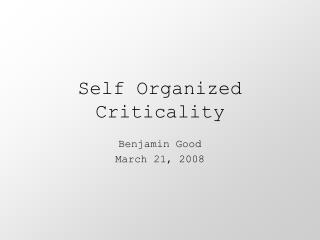Download PresentationSelf Organized Criticality

# Self Organized Criticality - PowerPoint PPT PresentationDownload Presentation## Self Organized Criticality

- - - - - - - - - - - - - - - - - - - - - - - - - - - E N D - - - - - - - - - - - - - - - - - - - - - - - - - - -
##### Presentation Transcript

1. Self Organized Criticality Benjamin Good March 21, 2008

2. What is SOC? • Self Organized Criticality (SOC) is a concept first put forth by Bak, Tang, and Wiesenfeld (all theoretical physicists) in their famous 1988 paper. • SOC models have been applied to earthquakes, evolution, neuron-processes, quantum gravity, and many other areas. • It centers around two key concepts: Criticality: a concept from statistical physics characterized by a lack of a characteristic time/length scale, fractal behavior, and power laws.Self Organized: this critical state arises naturally, regardless of initial conditions, rather than requiring exactly tuned parameters.

3. Characteristics of Criticality • Divergence of the correlation length ξ introduced in Yeomans’ book. • Certain observables (e.g. distribution of patch sizes) obey power laws • Universality – extremely different systems display the same behavior regardless of their dynamical rules. • System is often sensitive to small perturbations. • However, criticality is usually obtained by finely tuning a parameter (e.g. temperature for phase transitions), so they would be unlikely to naturally arise.

4. The BTW Model: Sand Piles • The original SOC model was based on sand piles. • Sand piles can reach a “critical state” where the addition of just one more piece of sand can trigger an avalanche of any size. • The concept of universality ensures that studying this model can tell us much about other processes, despite the silly nature of the particular example.

5. BTW Model in 1-D • There are N points on a line, and the height of sand at a point x is given by h(x). • The slope, z(x), at a point x is then given by z(x) = h(x) – h(x)+1 • To add a piece of sand at a point x, we take: z(x) = z(x)+1 z(x-1) = z(x-1)-1 • If the slope at any one point is higher than some critical value zc, a piece of sand fallsdown: z(x) = z(x) -2 z(x±1) = z(x±1)+1

6. BTW Model in 1-D • The pile is stable if z(x) ≤ zc for all x, so there are zcN stable configurations. • If sand is added randomly, the system will reach the minimally stable state (z(x) = zc for all x). If an extra piece of sand is added, it just falls all the way down the pile and off the edge. • Thus, no interesting behavior in the 1-Dimensional case. SOC is not present.

7. BTW Model in 2-D • To see interesting behavior, the model must have D ≥ 2. • In two dimensions, the height is given by h(x,y) and the slope by z(x,y) = 2h(x,y)-h(x+1)-h(x,y+1) • Our rules new 2-D rules become:Adding: z(x,y) = z(x,y)+2 z(x-1,y)=z(x-1,y)-1 z(x,y-1)=z(x,y-1)-1Falling: z(x,y) = z(x,y) – 4 z(x ± 1,y) = z(x ± 1, y)+1 z(x,y ± 1) = z(x, y ± 1)+1

8. BTW Model in 2-D • Will the system evolve towards the minimally stable state again? • NO! Because each point is connected to more than one other point, a small perturbation amplifies and travels throughout the entire pile. • Thus, the minimally stable state is unstable with respect to small fluctuations and cannot be an “attractor” of the dynamics.

9. BTW Model in 2-D • As the system evolves, more and more “more than critically stable” patches arise and will impede the motion of the perturbation. • Thus, the pile seems to be in a critical state, and since it arose on its own, it is a self organized critical state. Now we start looking for power laws.

10. Power Laws and Avalanche Sizes • Critical states are slightly perturbed and the resulting avalanches are measured. • We then form a distribution of avalanche sizes D(s) and try to fit it to a power law.

11. 1/f Noise • The critical sandpile also displays a phenomenon called 1/f noise. • This means that its power spectrum, defined by follows the form S(f) = 1/fb for b≈1. • This differs from random “white noise”, which is given by 1/f0 and that of a random (Brownian) walk, which is given by 1/f2. 1/f noise is usually defined as anything with 0<b<2

12. Applications to evolution • Many researchers (including Bak himself) have been inspired to apply SOC concepts to evolution. • This was prompted by several studies that discovered power law-like behavior in extinctions, evolutionary activity, etc. • SOC models are appealing in this context, because they offer a natural explanation for how these phenomena arose (they self organized), whereas many existing models yield the desired power laws only if certain parameters are tuned. • However, much of the justification for SOC models is based only on the fact that power laws are observed.

13. Are power laws enough evidence for SOC? • Newman, in a 1995 paper on the evidence for SOC in evolution, asks whether power laws are actually present in the data and whether this is enough to imply SOC in evolutionary processes. • Results: although the evidence for power laws is good, additional non-SOC mechanisms could account for them (e.g. environmental stresses).